# Code examples¶

## Bar plot examples¶

examples.barPlots.example1_simple(saveFiles_)[source]

In this example, a simple bar plot is created: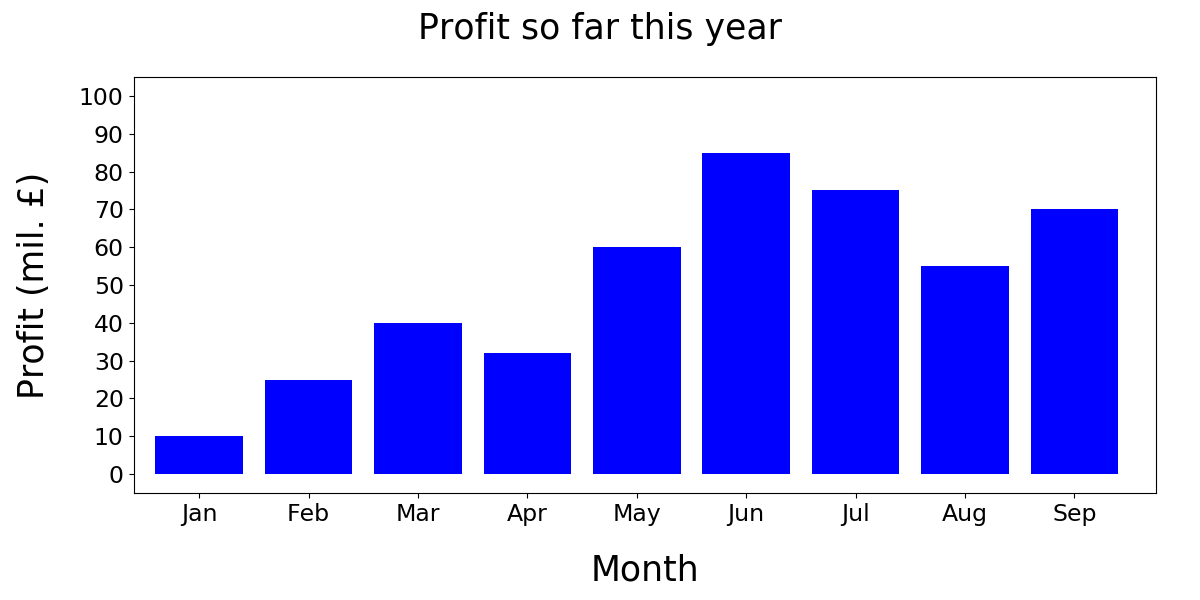Parameters: saveFiles – Boolean if True, figures are saved, if False, figures are displayed
examples.barPlots.example2_errorBars(saveFiles_)[source]

In this example, a multi-bar plot with error bars is created. A legend with a title and horizontal-only grid is shown: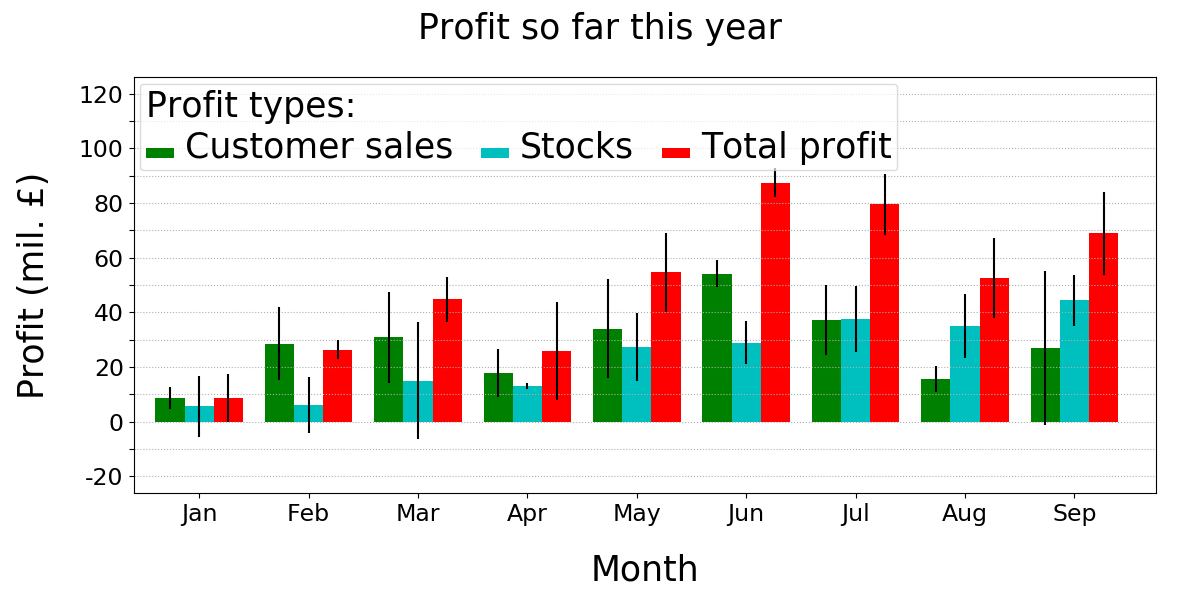Parameters: saveFiles – Boolean if True, figures are saved, if False, figures are displayed

## Line and box plot examples¶

examples.linePlots.example1_simple(saveFiles_)[source]

In this example, a single-line plot is created: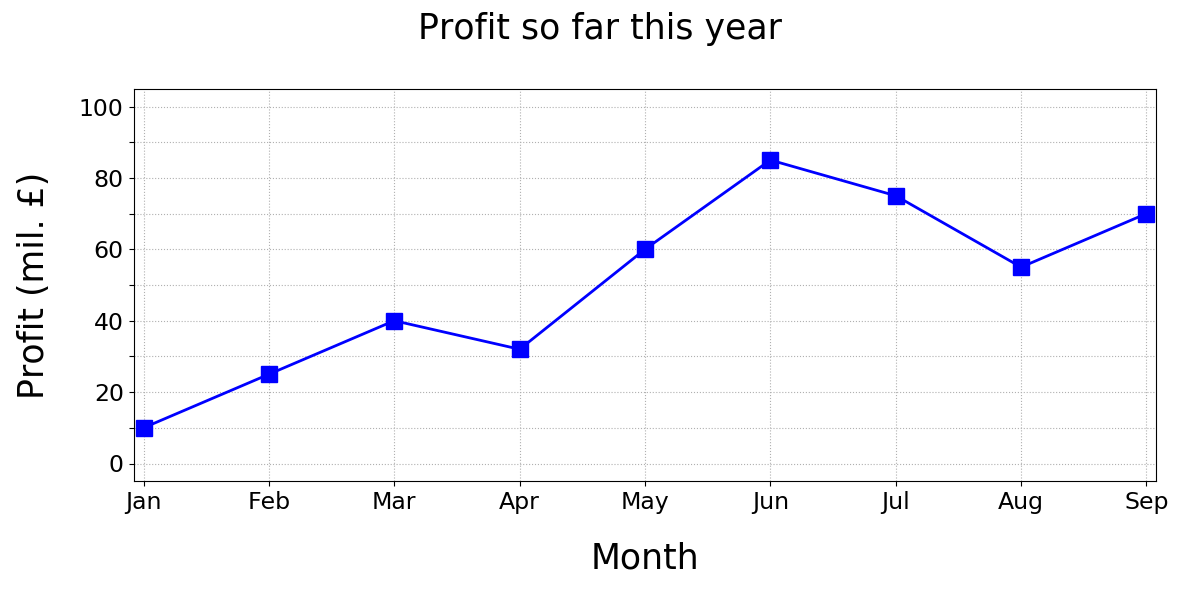Parameters: saveFiles – Boolean if True, figures are saved, if False, figures are displayed
examples.linePlots.example2_multiple(saveFiles_)[source]

In this example, a multi-line plot is created and its custom colours and markers are specified.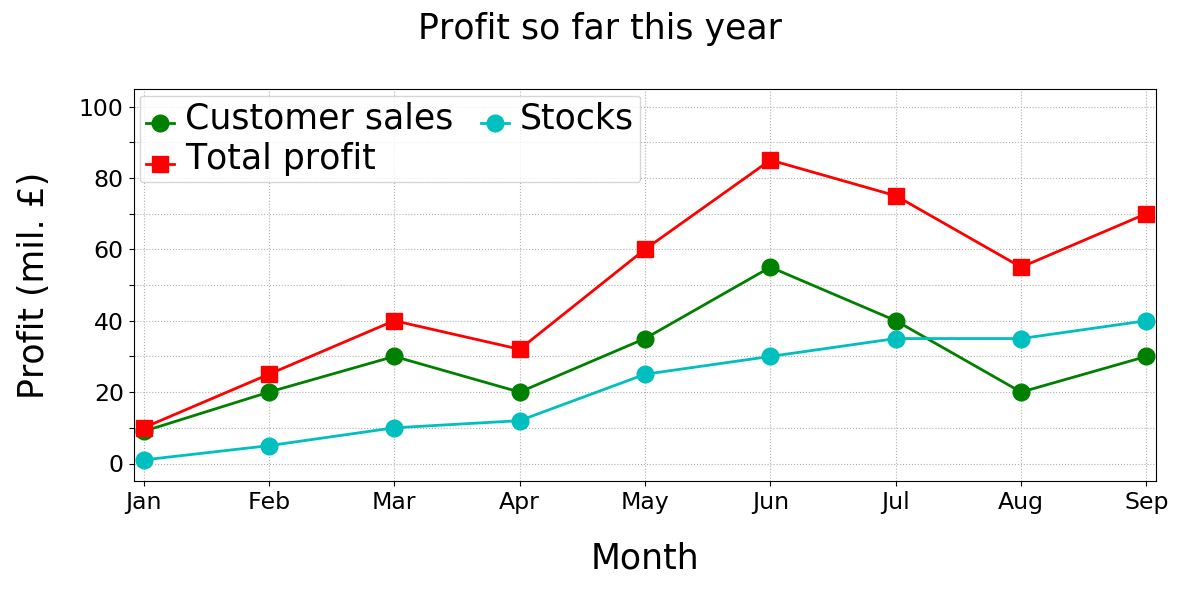Parameters: saveFiles – Boolean if True, figures are saved, if False, figures are displayed
examples.linePlots.example3_errorBars(saveFiles_)[source]

In this example, a multi-line plot with error bars is created, with horizontal-only grid shown and with data points connected together based on which year quarter they belong to: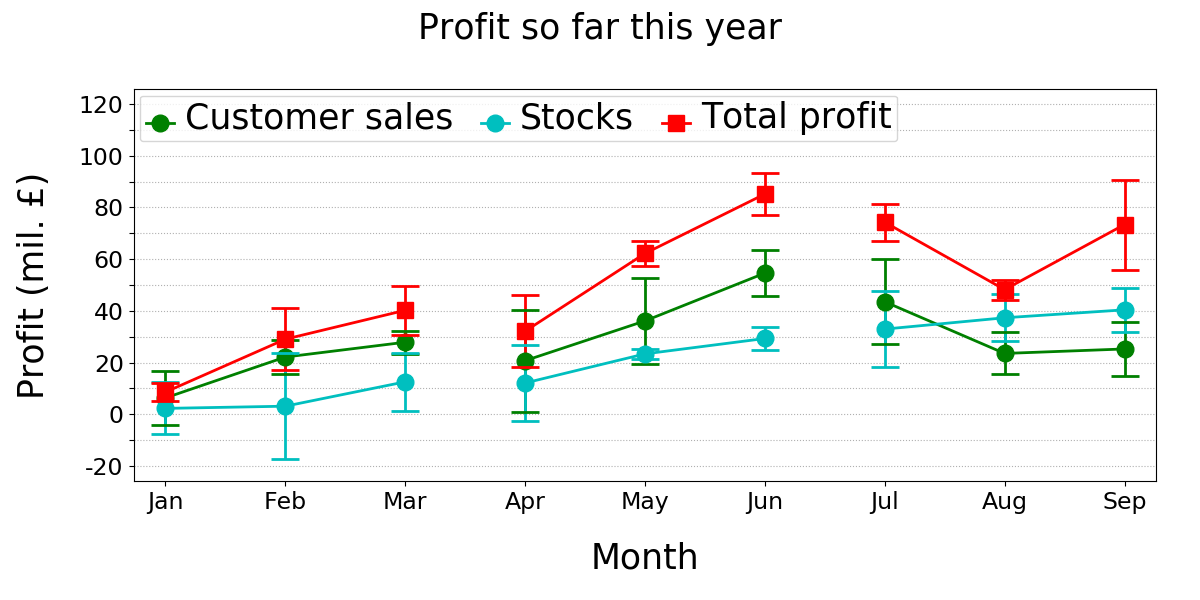Parameters: saveFiles – Boolean if True, figures are saved, if False, figures are displayed
examples.linePlots.example4_boxPlots(saveFiles_)[source]

In this example, a multi-line plot with box plots is created, without the grid or data point connections shown.

Also, a Willcoxon test is performed find out data points with significant differences between them. A * notation is used next to a month where there is significant difference with p=0.05. A ** notation is used when there is a significant different with p=0.01.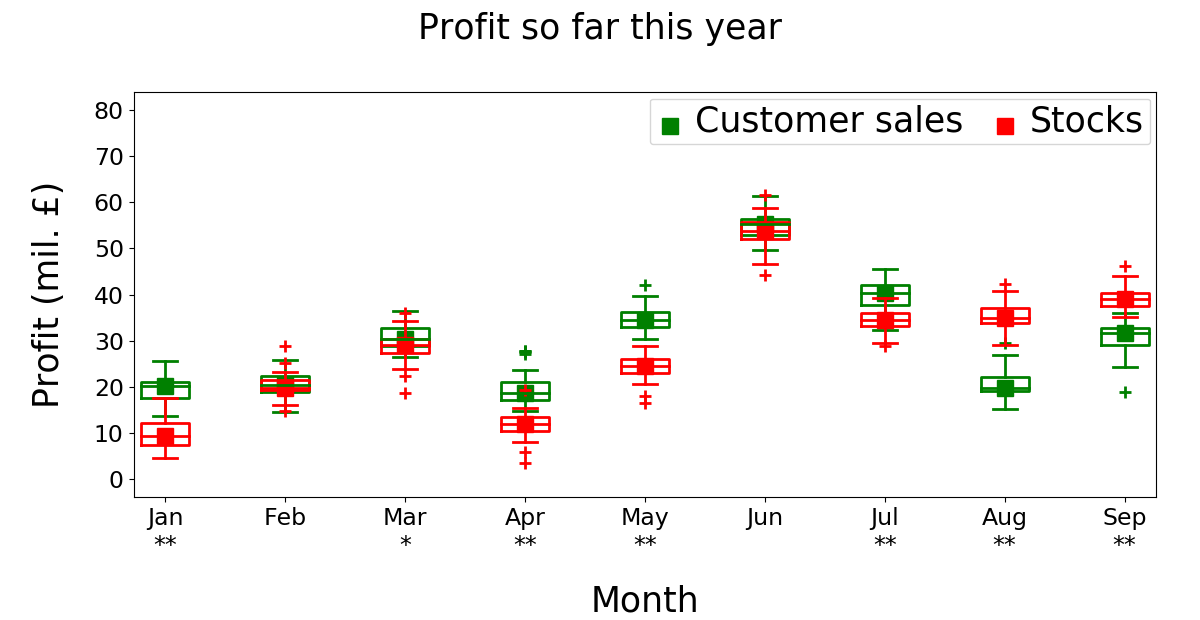Parameters: saveFiles – Boolean if True, figures are saved, if False, figures are displayed

## Matrix plot examples¶

examples.matrixPlots.example1_simple(saveFiles_)[source]

In this example, a simple matrix plot created: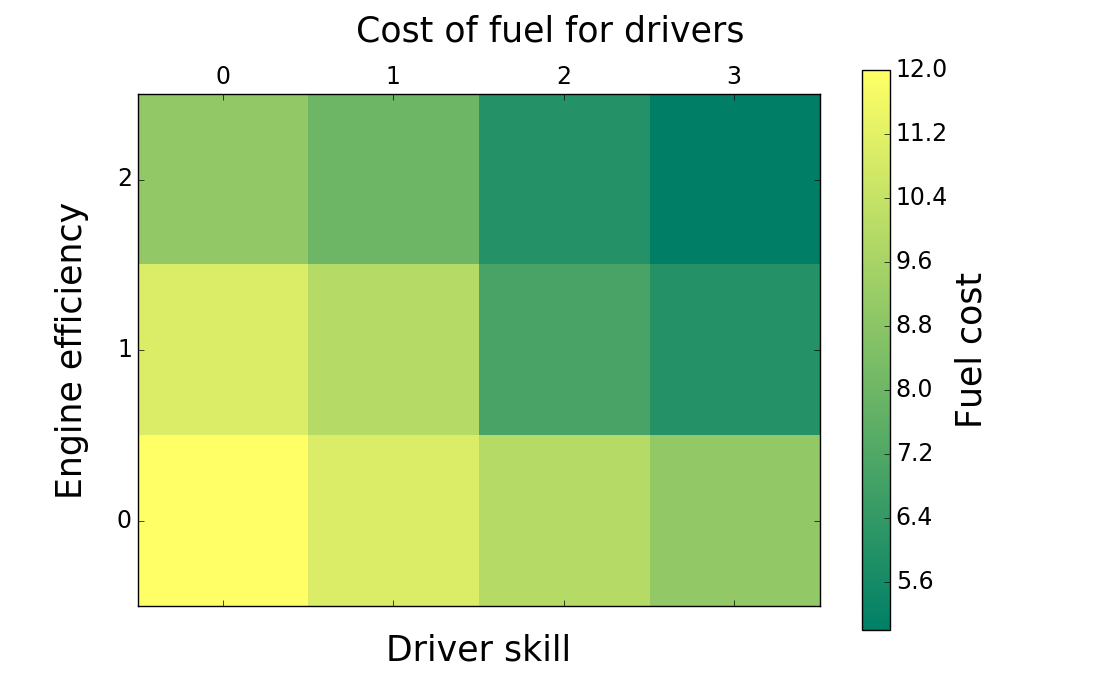Parameters: saveFiles – Boolean if True, figures are saved, if False, figures are displayed
examples.matrixPlots.example2_customAnnotationsAndColorMap(saveFiles_)[source]

In this example, a matrix plot with custom annotations and color map is created: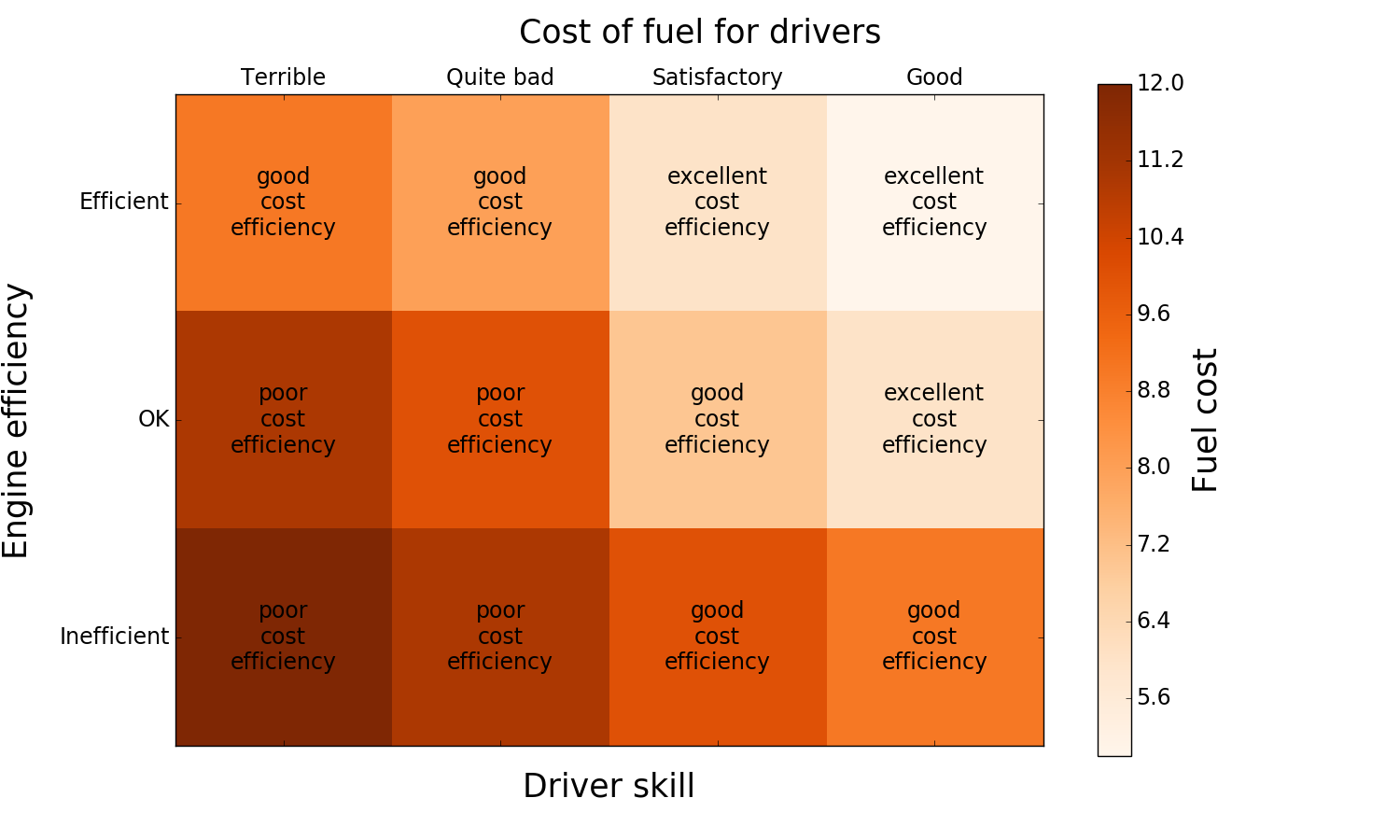Parameters: saveFiles – Boolean if True, figures are saved, if False, figures are displayed

## Pie chart examples¶

examples.pieCharts.example1_simple(saveFiles_)[source]

In this example, a simple pie chart is created: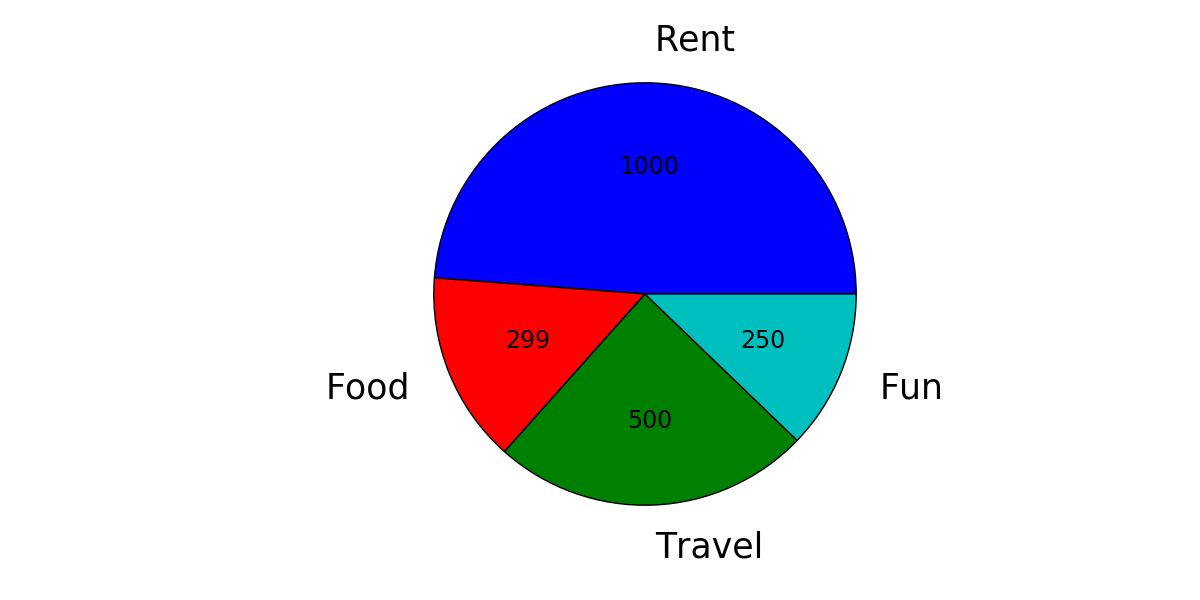Parameters: saveFiles – Boolean if True, figures are saved, if False, figures are displayed
examples.pieCharts.example2_colors(saveFiles_)[source]

In this example, the title, colors and font sizes are specified. Percentage values and actual values are also shown: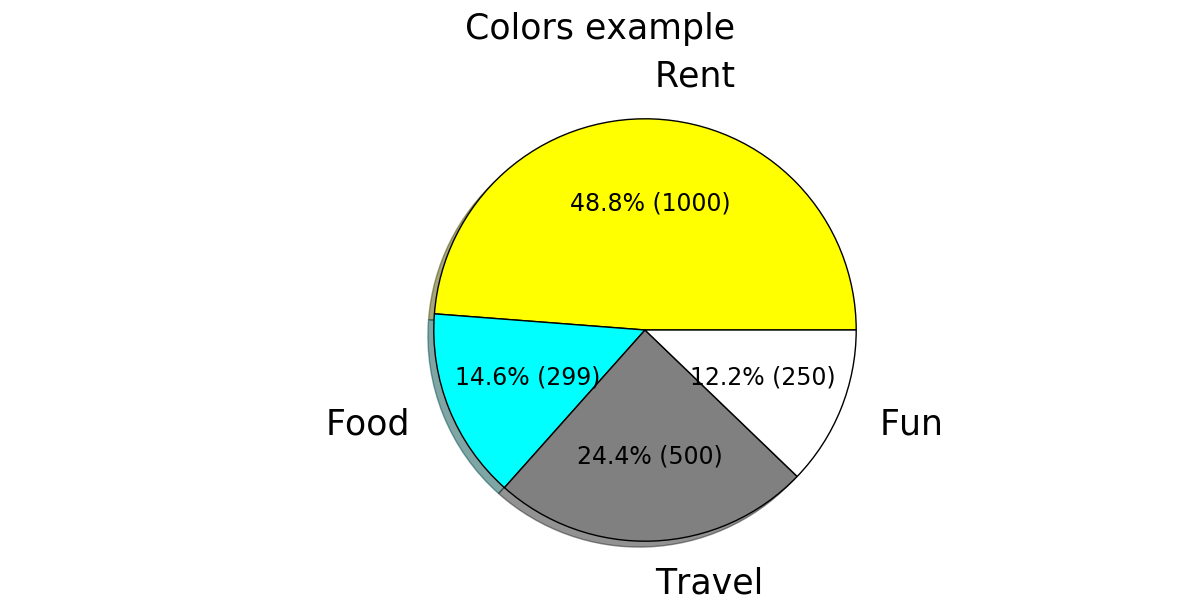Parameters: saveFiles – Boolean if True, figures are saved, if False, figures are displayed
examples.pieCharts.example3_multiFigure(saveFiles_)[source]

In this example, two figures are created below each other and saved into a single file: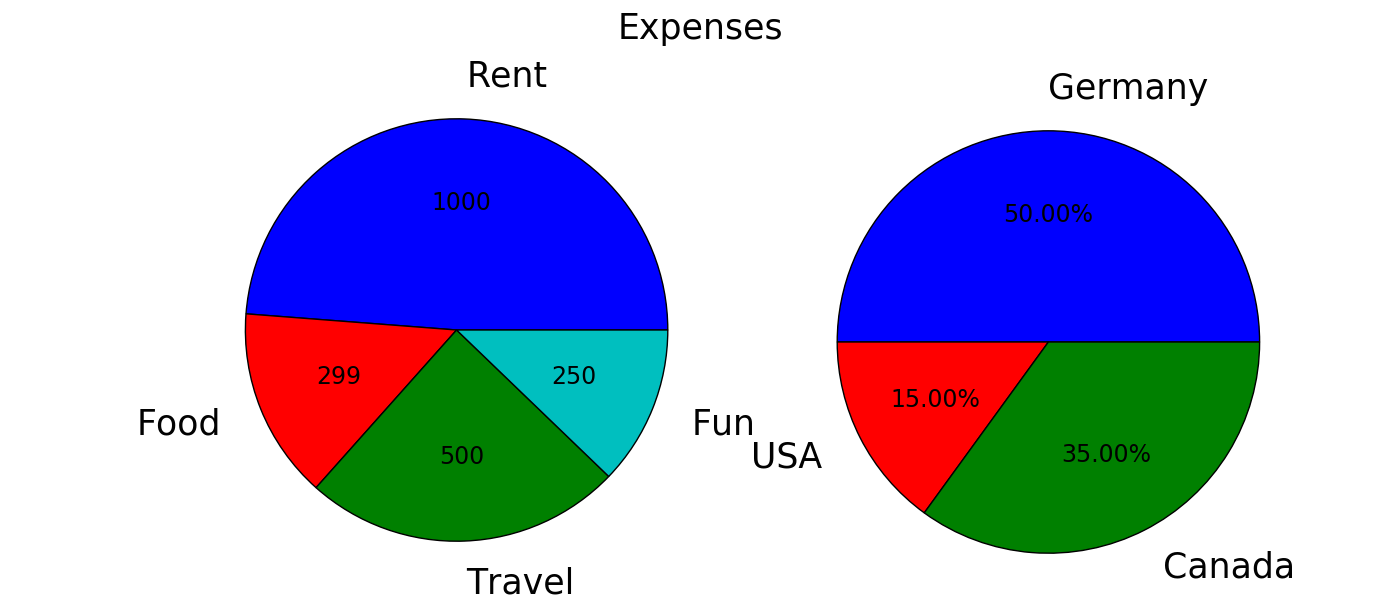Parameters: saveFiles – Boolean if True, figures are saved, if False, figures are displayed